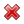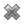# Fight Finance

#### CoursesTagsRandomAllRecentScoresScoreskeithphw $6,011.61 Jade$1,815.80 Chu $789.98 royal ne...$750.00 Leehy $713.33 Visitor$650.00 JennyLI $625.61 Visitor$590.00 ZOE HY $560.00 Visitor$555.33 Visitor $550.00 Visitor$550.00 Visitor $540.00 Visitor$500.00 Yizhou $489.18 Visitor$480.00 Visitor $464.70 Visitor$460.00 Visitor $460.00 LWH$460.00

For an asset price to double every 10 years, what must be the expected future capital return, given as an effective annual rate?

For an asset price to triple every 5 years, what must be the expected future capital return, given as an effective annual rate?

How many years will it take for an asset's price to double if the price grows by 10% pa?

How many years will it take for an asset's price to quadruple (be four times as big, say from $1 to$4) if the price grows by 15% pa?

For an asset's price to quintuple every 5 years, what must be its effective annual capital return? Note that a stock's price quintuples when it increases from say $1 to$5.

How many years will it take for an asset's price to triple (increase from say $1 to$3) if it grows by 5% pa?

Question 906  effective rate, return types, net discrete return, return distribution, price gains and returns over time

For an asset's price to double from say $1 to$2 in one year, what must its effective annual return be? Note that an effective annual return is also called a net discrete return per annum. If the price now is $P_0$ and the price in one year is $P_1$ then the effective annul return over the next year is:

$$r_\text{effective annual} = \dfrac{P_1 - P_0}{P_0} = \text{NDR}_\text{annual}$$

For an asset's price to double from say $1 to$2 in one year, what must its continuously compounded return $(r_{CC})$ be? If the price now is $P_0$ and the price in one year is $P_1$ then the continuously compounded return over the next year is:

$$r_\text{CC annual} = \ln{\left[ \dfrac{P_1}{P_0} \right]} = \text{LGDR}_\text{annual}$$

Question 908  effective rate, return types, gross discrete return, return distribution, price gains and returns over time

For an asset's price to double from say $1 to$2 in one year, what must its gross discrete return (GDR) be? If the price now is $P_0$ and the price in one year is $P_1$ then the gross discrete return over the next year is:

$$\text{GDR}_\text{annual} = \dfrac{P_1}{P_0}$$

For a share price to double over 7 years, what must its capital return be as an effective annual rate?